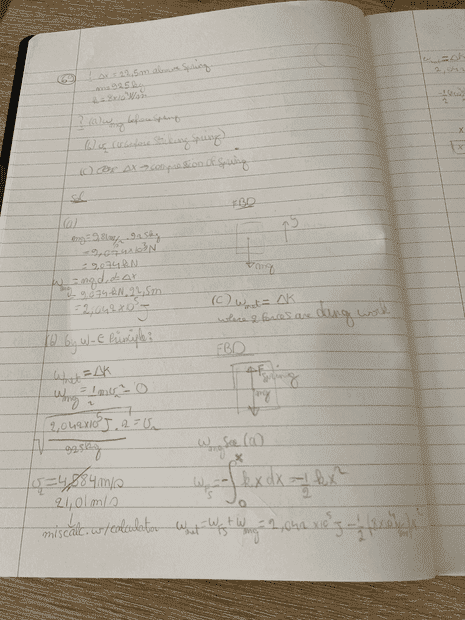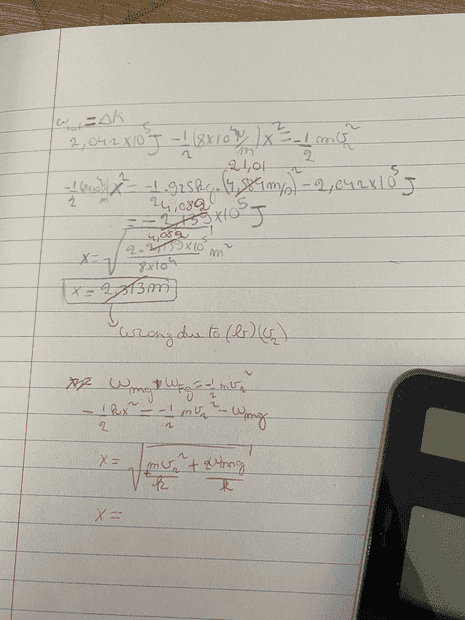# Work done on a falling elevator by a spring + gravity

• simphys
In summary, the problem is that the second part of the motion has an incorrect initial velocity, and the final velocity should be zero.f

#### simphys

Homework Statement
An elevator cable breaks when a 925-kg elevator
is 22.5 m above the top of a huge spring
at the bottom of the shaft. Calculate
(a) the work done by gravity on the elevator before it hits the
spring; (b) the speed of the elevator just before striking the
spring; (c) the amount the spring compresses (note that here
work is done by both the spring and gravity).
Relevant Equations
WE principle + w=Fd
I don't understand what I have done wrong in part (c) I have the initial velocity for the second part of the motion and have the final velocity zero and then the net work done is W_mg + W_Fs and the actual answer for x is 2.37m

Here is my solutionsmall edit: After getting V_2 = 21m/s, x = 3.19m and the ,(comma) is a decimal point here! .(dot) is multiplication

Last edited:
•Delta2
I think I see the problem.. I used the work done by gravity from the free fall motion part.
for the second part it actually is W_mg = mgx (if I am not mistaking)

I got the following: ##-4E4*x^2 + 9.074E3*x + 2.04E5=0 ##
which I solved with the calculator directly, but using the quadractic formula would be a lot of writing, is there another way I can look at this problem or something?

I got the following: ##-4E4*x^2 + 9.074E3*x + 2.04E5=0 ##
which I solved with the calculator directly, but using the quadractic formula would be a lot of writing, is there another way I can look at this problem or something?
I cannot read the value of k in the posted image, but I deduce it must be 8E4 N/m.

•simphys
I cannot read the value of k in the posted image, but I deduce it must be 8E4 N/m.
Thank you! I thought that there may be a way to just use the initial and final position instead of breaking it up into part, but I didn't try. I supposed that it might've work that is why I asked.

Thank you! I thought that there may be a way to just use the initial and final position instead of breaking it up into part, but I didn't try. I supposed that it might've work that is why I asked.
•